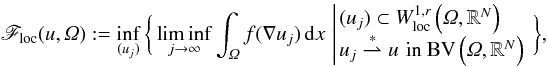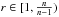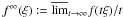Home

# Relaxation in BV of integrals with superlinear growth

## Abstract

We study properties of the functional

\begin{eqnarray} \mathscr{F}_{{\rm loc}}(u,\Omega):= \inf_{(u_{j})}\bigg\{ \liminf_{j\rightarrow\infty}\int_{\Omega}f(\nabla u_{j})\ud x\, \left| \!\!\begin{array}{rl} & (u_{j})\subset W_{{\rm loc}}^{1,r}\left(\Omega, \RN\right) \\ & u_{j}\tostar u\,\,\textrm{in }\BV\left(\Omega, \RN\right) \end{array} \right. \bigg\}, \end{eqnarray}$\begin{array}{ccc}{F}_{\mathrm{loc}}\mathrm{\left(}\mathit{u,\Omega }\mathrm{\right)}\mathrm{:}\mathrm{=}\underset{\mathrm{\left(}{\mathit{u}}_{\mathit{j}}\mathrm{\right)}}{\mathrm{inf}}\left\{\right\\underset{\mathit{j}\mathrm{\to }\mathrm{\infty }}{\mathrm{lim}\mathrm{inf}}{\mathrm{\int }}_{\mathit{\Omega }}\mathit{f}\mathrm{\left(}\mathrm{\nabla }{\mathit{u}}_{\mathit{j}}\mathrm{\right)}\mathrm{d}\mathit{x}\left|\begin{array}{c}\\ & \\ & \end{array}\right\left\}\right\\mathit{,}& & \end{array}$
where u ∈ BV(Ω;RN), and f:RN × n → R is continuous and satisfies 0 ≤ f(ξ) ≤ L(1 + | ξ | r). For r ∈ [1,2), assuming f has linear growth in certain rank-one directions, we combine a result of [A. Braides and A. Coscia, Proc. Roy. Soc. Edinburgh Sect. A 124 (1994) 737–756] with a new technique involving mollification to prove an upper bound for Floc. Then, for \hbox{$r\in[1,\frac{n}{n-1})$}$\mathit{r}\mathrm{\in }\mathrm{\left[}\mathrm{1}\mathit{,}\frac{\mathit{n}}{\mathit{n}\mathrm{-}\mathrm{1}}\mathrm{\right)}$ , we prove that Floc satisfies the lower bound
\begin{equation*} \scF_{{\rm loc}}(u,\Omega) \geq \int_{\Omega} f(\nabla u (x))\ud x + \int_{\Omega}\finf \bigg(\frac{D^{s}u}{|D^{s}u|}\bigg)\,|D^{s}u|, \end{equation*}${F}_{\mathrm{loc}}\mathrm{\left(}\mathit{u,\Omega }\mathrm{\right)}\mathrm{\ge }{\mathrm{\int }}_{\mathit{\Omega }}\mathit{f}\mathrm{\left(}\mathrm{\nabla }\mathit{u}\mathrm{\left(}\mathit{x}\mathrm{\right)}\mathrm{\right)}\mathrm{d}\mathit{x}\mathrm{+}{\mathrm{\int }}_{\mathit{\Omega }}{\mathit{f}}^{\mathrm{\infty }}\left(\right\frac{{\mathit{D}}^{\mathit{s}}\mathit{u}}{\mathrm{|}{\mathit{D}}^{\mathit{s}}\mathit{u}\mathrm{|}}\left)\right\mathrm{|}{\mathit{D}}^{\mathit{s}}\mathit{u}\mathrm{|}\mathit{,}$
provided f is quasiconvex, and the recession function f (defined as \hbox{$f^{\infty}(\xi):= \overline{\lim}_{t\rightarrow\infty}f(t\xi )/t$}${\mathit{f}}^{\mathrm{\infty }}\mathrm{\left(}\mathit{\xi }\mathrm{\right)}\mathrm{:}\mathrm{=}{\overline{)\mathrm{lim}}}_{\mathit{t}\mathrm{\to }\mathrm{\infty }}\mathit{f}\mathrm{\left(}\mathit{t\xi }\mathrm{\right)}\mathit{/}\mathit{t}$ ) is assumed to be finite in certain rank-one directions. The proof of this result involves adapting work by [Kristensen, Calc. Var. Partial Differ. Eqs. 7 (1998) 249–261], and [Ambrosio and Dal Maso, J. Funct. Anal. 109 (1992) 76–97], and applying a non-standard blow-up technique that exploits fine properties of BV maps. It also makes use of the fact that Floc has a measure representation, which is proved in the appendix using a method of [Fonseca and Malý, Annal. Inst. Henri Poincaré Anal. Non Linéaire 14 (1997) 309–338].

Hide All

# Relaxation in BV of integrals with superlinear growth

## Metrics

### Full text viewsFull text views reflects the number of PDF downloads, PDFs sent to Google Drive, Dropbox and Kindle and HTML full text views.

Total number of HTML views: 0
Total number of PDF views: 0 *Loading metrics...

### Abstract viewsAbstract views reflect the number of visits to the article landing page.

Total abstract views: 0 *Loading metrics...

* Views captured on Cambridge Core between <date>. This data will be updated every 24 hours.

Usage data cannot currently be displayed.